Calibration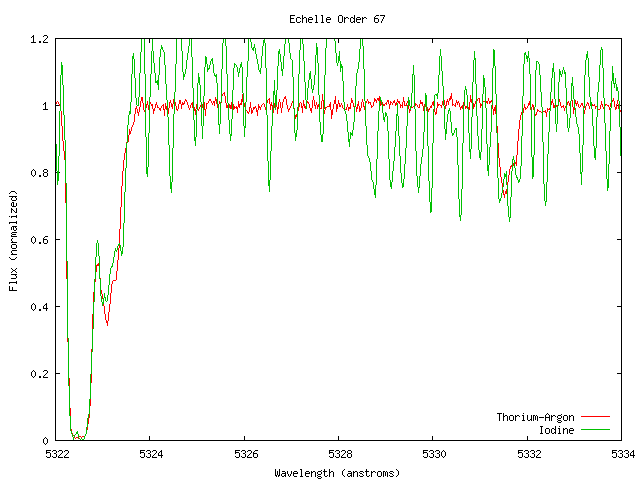The absorption of Iodine is much weaker than FeII near 5322 but equally strong near 5332. Looking at the absorption of FeII near 5332 the Thorium-Argon line has a clear characteristic shape whereas the Iodine line is not well defined.

# The Predicament

Though we have many more calibration points using Iodine, the strength of the Iodine lines is comparable to some of the absorption lines1. Thorium- Argon has too few lines to calibrate with, resulting in a "fuzziness" of how we measure. Iodine has too many lines, producing a "fuzziness" of what we are measuring. In other words, we either have a bad ruler to measure a clear point or a blurred point to measure with a good ruler. Though the data taken with Thorium-Argon can be indirectly calibrated by the Iodine data, the precision will be somewhat reduced. The result may lead to only a slightly more precise variation in alpha then previously measured.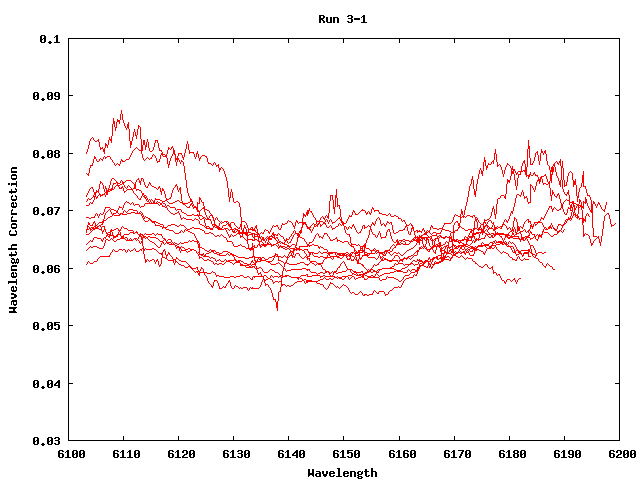Jonathan shows that the wavelengths should be shifted on the order of up to one tenth of an angstrom. Each run tends to be tightly grouped whereas between each run there is an appreciable shift. How to read: Run 4-1 was recorded Oct 4th and is the 2nd run that day (starts with zero instead of one). Only data with Iodine, labeled I2 in the table, is plotted here.

# Calibrating Wavelength with Iodine Data

In order to calibrate, the data collected by HIRES with the Iodine cell in place had to be compared to laboratory measurements of the Iodine spectrum. Each pixel on the HIRES camera is associated with a particular wavelength. Due to the complicated path light travels inside the telescope and the digitized binning determined by the number of camera pixels, the data is not perfectly precise. Since the laboratory Iodine measurements are more precise than the resolution of HIRES, their wavelengths are shifted to fit the data of HIRES with the Iodine cell. These shifts are subtracted from the wavelengths of the HIRES data for each individual run and Echelle order. For an in-depth look into the process of calculating the wavelength shifts, check out Jonathan's writeup on Iodine Calibration.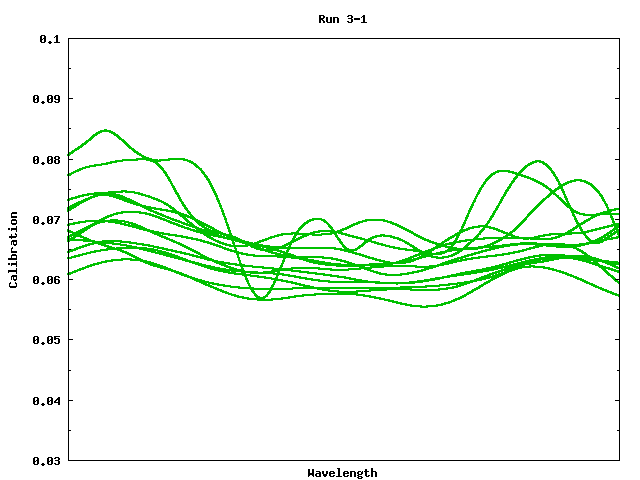Whereas the previous image shows the direct results of the calibration, this image shows the weighted cubic spline that runs through the calibration points. This spline provides the best approximation for each shift per run. Lastly, the x-axis represents the wavelength but remains blank since the Echelle orders (which have different wavelengths) are overlaid.

# Smoothing the Calibrations

To find the wavelength shift, Jonathan's convolution program sampled over several HIRES data points to produce one calibration point. Through this use of sampling Jonathan also derived the error in the calibrations. The function describing the calibration is far from analytic and requires a numerical approximation to correlate data points with the proper shift. Without a continuous line to describe the calibration there is no way to find precisely how much to shift the HIRES data. Utilizing the error to weight the calibration points, a cubic spline is used to fit a smooth line to these points. Though each calibration shift corresponds to several HIRES data points, this fitted line provides the ability to find shifts that correspond to each individual HIRES data point.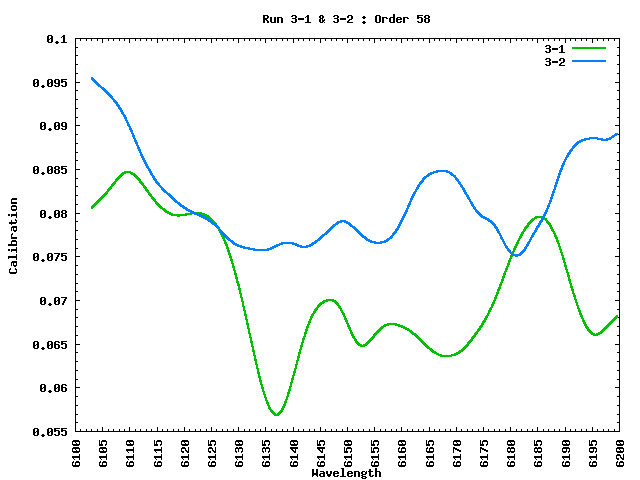This plot groups the calibration shift by day and order to show the possible trends hidden in the data.

# Looking For Trends in the Calibration Data

It is clear from the above image that the calibration shifts are spread out yet they appear to be well grouped for each run. While each individual run is precise, there is a non-negligible shift from one run to the next. There also appears to be a trend for runs performed on the same day but how much and why it varies has not been determined as of yet. It appears feasible to increase the precision of the Thorium-Argon data by utilizing the Iodine runs for that day. For instance Run 3-0 can be calibrated by Run 3-1 & 3-2 data as Run 5-1 can be calibrated by Run 5-0 & 5-3. By looking at the expandable "Table of Runs", the scheme of the runs should easily be understood.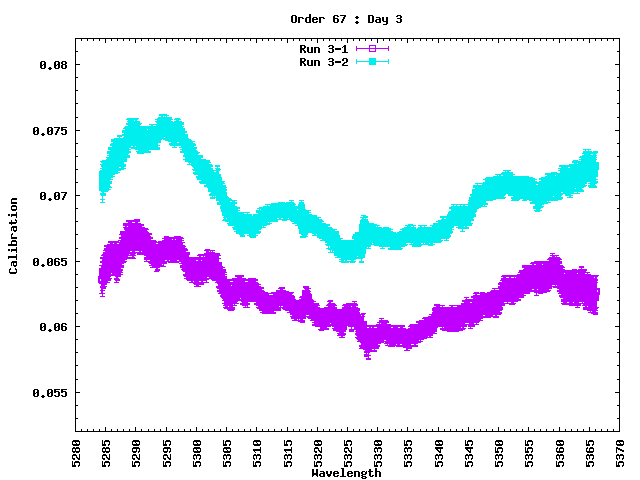First, the raw data with y-error bars is displayed for day 3. Second, the averaged data with y-error bars is inserted. Third, the data sets are approximated with a weighted cubic spline. Fourth, the averaged spline splits and moves towards being equally spaced. Lastly, the calibrated splines are labeled for Run 3-0 & 3-3.

# Calibrate Thorium-Argon w/ Iodine

As shown above, there is a definite trend within the calibration data with respect to day and Echelle order. For days 3 & 4, the first and last measurements use Thorium- Argon whereas the two in-between use Iodine (i.e. 3-1 & 3-2). On day 5, the first and fourth measurements use Iodine (i.e. 5-0 & 5-3) with Thorium-Argon used second, third, and fifth (see the above Table of Runs). The grouping of data appears to be determined by the order in which each run is measured as shown by the fact that days 3 & 4 have tightly grouped data and day 5 has a wider gap. In order to calibrate the Thorium-Argon Data, we created a template and adjusted it for each individual day and order as described in the graph to the side.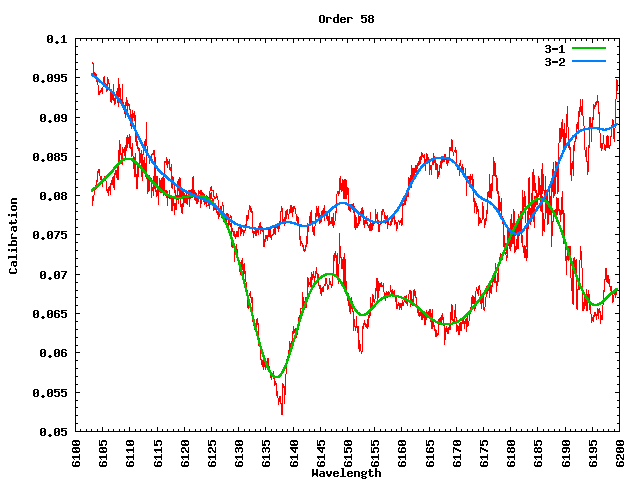The calculated calibration points appear scattered but a cubic spline smooths out the noise.

# Remove the Iodine lines from the Iodine data

### Work in Progress

Another solution is to use the calibrated Iodine data by removing the Iodine lines to show the "naked" absorption lines. There are a few questions that need to be analyzed first. Do the absorption lines and Iodine lines simply add linearly or is it a higher order process? How do we create a template of sorts to subtract off the Iodine lines? My naive intuition is that they do not simply add but that the linear term still dominates, making it a reasonable approximation. To perform the removal, the Iodine runs should first be splined correctly and then calibrated2. Next, use the precise Iodine data used in the calibration as the subtraction template3.